# Parallel Decoding for Random Linear Network Codes

### Created : August 16, 2021

This week I was exploring how parallelism can be incorporated into decoding of Random Linear Network Codes ? During decoding of RLNC coded pieces it's required to invert one N x N coding coefficient matrix, where N = #-of pieces coded together i.e. generation size. And matrix inversion is quite expensive O(N ** 3), which is why I'm interested in implementing faster decoding algorithm in kodr, a RLNC library I've started writing sometime ago. Currently implemented decoding technique works in progressive mode i.e. as soon as a new coded piece is received, decoder attempts to compute Reduced Row Echelon Form of updated matrix ( read considering new coded piece ), which keeps pieces partially decoded. When N-th linearly independent piece is received last iteration of RREF calculation decodes whole data matrix & original pieces are revealed. This scheme works fine, but works in single threaded mode. So I'm looking for scheme which allows leveraging multiple CPU cores while decoding pieces, which are coded with large generation size ( read N is high ). As soon as generation size goes beyond 64, decoding speed starts to fall down very fast. I've found literatures around parallelization of RLNC decoding which also works in progressive mode i.e. decoder doesn't need to wait for receiving all of N-many coded pieces before it can start decoding those pieces.

Today I'll dig deep into Parallel Role Division Progressive Decoding technique, proposed here, which I'm already implementing in kodr. You may want to check implementation progress.

In single threaded RLNC decoding implementation, there exists only one thread of execution which accepts newly received coded pieces; places coding vector into coding coefficient matrix & coded data vector into coded data matrix; reduces coefficient matrix into row echelon form, also replicates respective row operations in coded data matrix. This flow of execution is followed by executor thread on reception of every new coded piece. This way prograssively coded pieces get decoded. Finally reception of N-th linearly independent piece reveals all original pieces, N = generation size.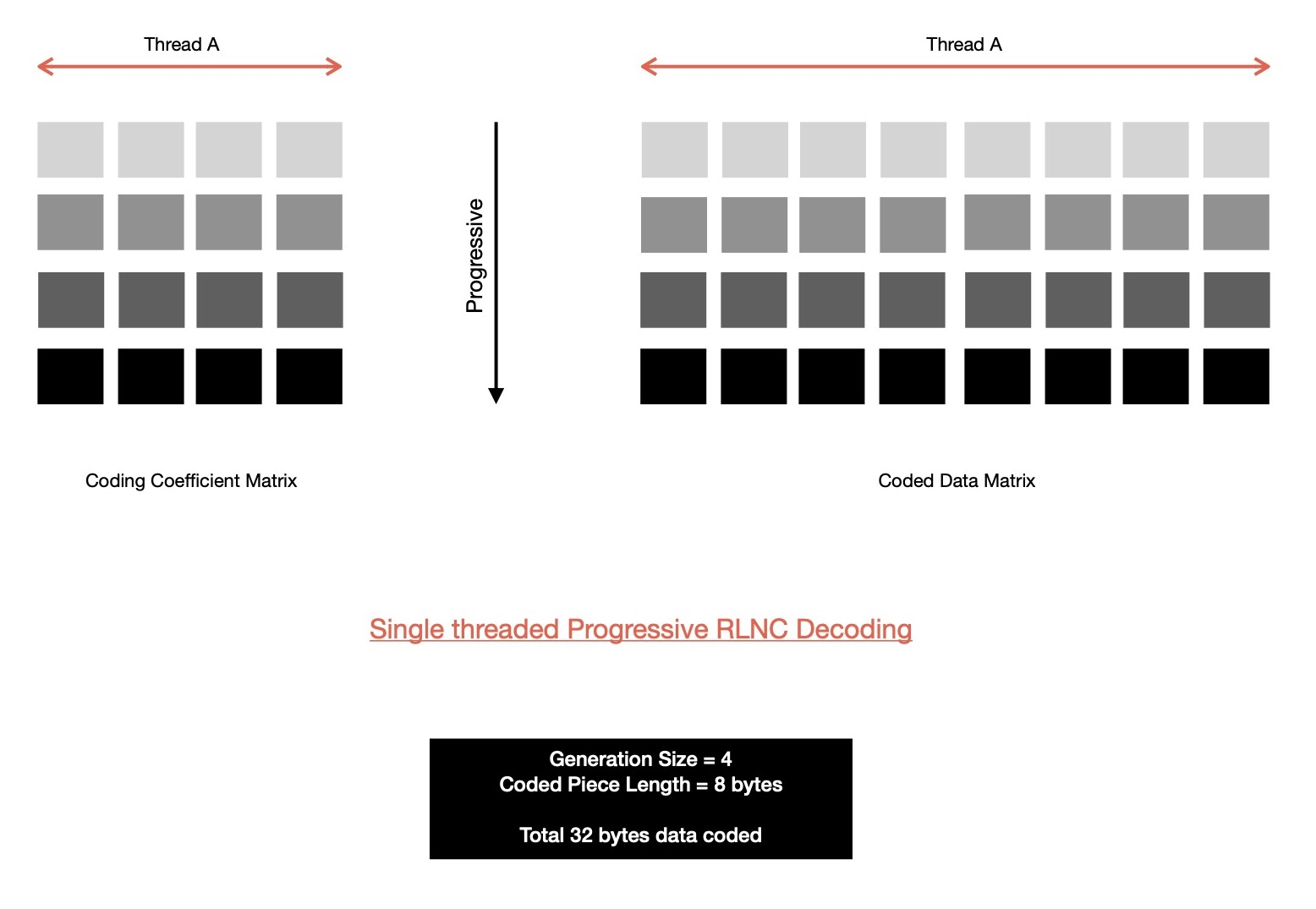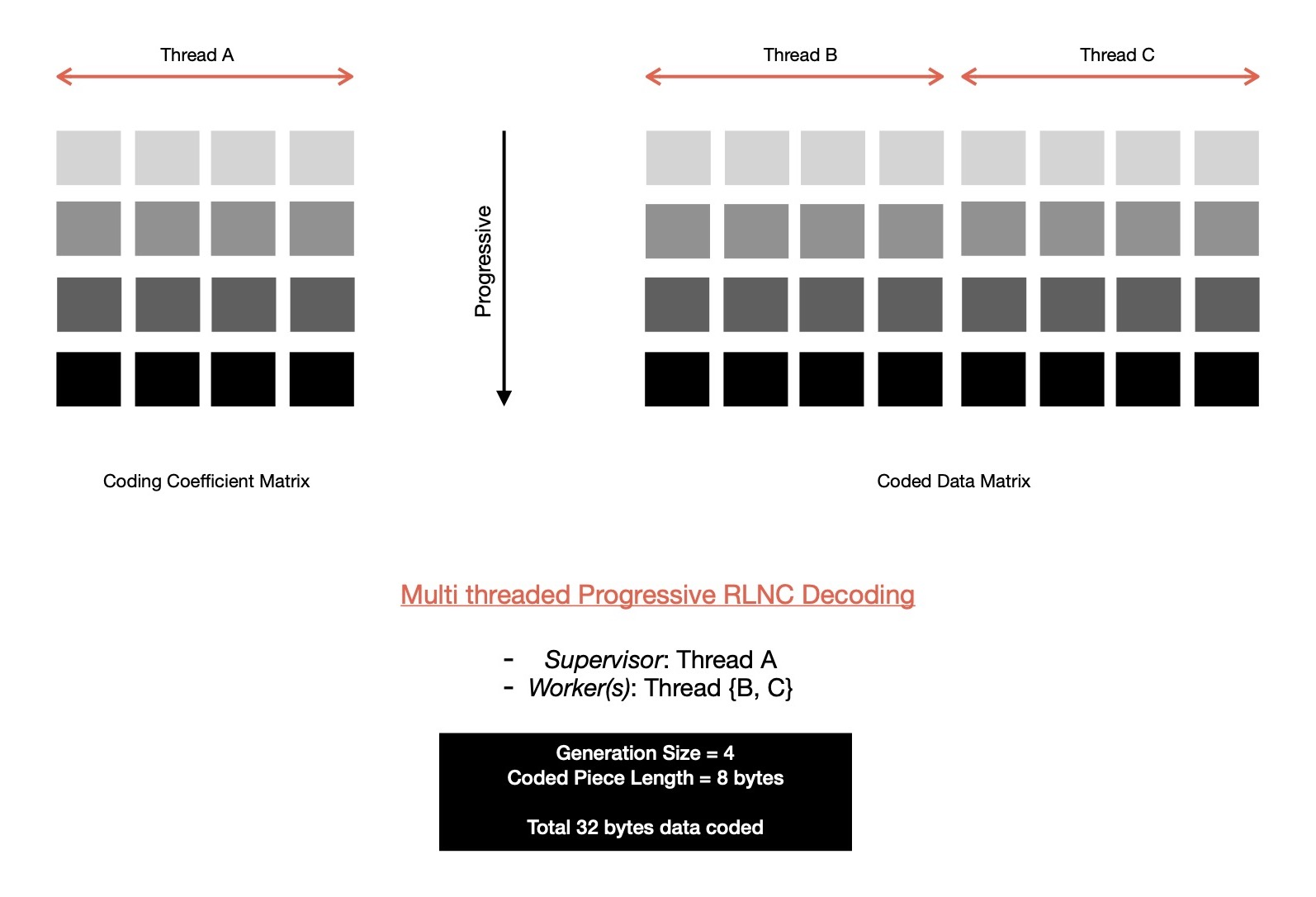Now I'm going to demonstrate whole parallel decoding process with the help of an example. Say, generation size is 3 i.e. 3 pieces to be coded together & each piece is of size 4 bytes. So decoder needs to receive 3 linearly independent coded pieces to be able successfully decode original pieces. Supervisor thread works on coding coefficient matrix of dimension K x 3, where K = [1..3] & 2 worker threads are responsible for managing two non-overlapping coded data submatrices, each of dimension K x 2, where K = [1..3].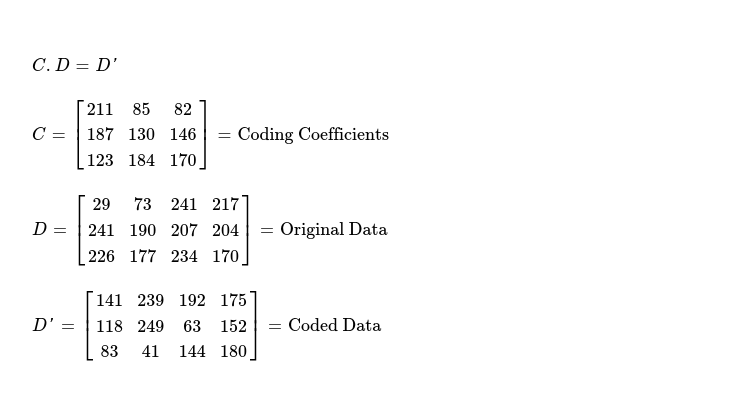After reception of every coded piece, three steps are performed in order on updated coding coefficient matrix by supervisor thread. And respective steps are also replicated on coded data matrix by two worker threads.

1. Forward elimination
2. Linear dependence test
3. Backward substitution

When first coded piece is received, supervisor thread finds it's the only row in coding coefficient matrix, which is why step {1, 3} can't be performed for this piece. Linear dependence check requires dividing row C by pivot element at C & it can be clearly understood that as this is the only piece received so it's indeed useful i.e. trivial case. Resulting coding coefficient matrix looks like.

\$ python3 >> import galois >> gf256 = galois.GF(2**8) >> gf256([211, 85, 82]) / gf256(211) GF([ 1, 153, 97], order=2^8)

Now same step needs to be performed on coded data matrix, by two worker threads. Both worker threads divide row D' of their respective submatrices by weight 211.

>> coded_data_row = [141, 239, 192, 175] >> worker_thread_1 = coded_data_row[:2] >> worker_thread_2 = coded_data_row[2:] >> worker_thread_1 / gf256(211) GF([124, 109], order=2^8) >> worker_thread_2 / gf256(211) GF([221, 38], order=2^8)

After reception of second coded piece, this is how coding coefficient matrix & coded data matrix looks like.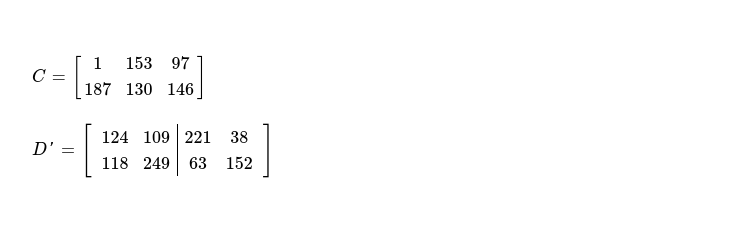Forward elimination cleans elements below pivots, so I multiply row C with weight at cell C; add resulting vector ( element by element ) with row C; finally put resulting vector in row C.

>> tmp = gf256([1, 153, 97]) * gf256(187) // multiply by C >> tmp GF([187, 170, 164], order=2^8) >> gf256([187, 130, 146]) + tmp // then add with C GF([ 0, 40, 54], order=2^8) // = C, after step 1

As element at pivot cell C is non-zero, elements of row C can be divided by C --- piece is linearly independent. After step 2, C of coding coefficient matrix looks like below.

>> gf256([ 0, 40, 54]) / gf256(40) // divide C by 40 GF([ 0, 1, 200], order=2^8) // = C, after step 2

Backward substitution cleans up elements above pivot elements. For eliminating elements above pivot element C, elements of row C are multipled by C; resulting vector is element-wise added with row C; finally resulting vector replaces C. This way both pivots C, C have zero-ed cells above & below them.

>> tmp = gf256([ 0, 1, 200]) * gf256(153) // multiply by C >> tmp GF([ 0, 153, 58], order=2^8) >> tmp + gf256([1, 153, 97]) // then add with C GF([ 1, 0, 91], order=2^8) // = C, after step 3

Supervisor thread managed coding coefficient matrix looks like below, after second linearly independent piece is received.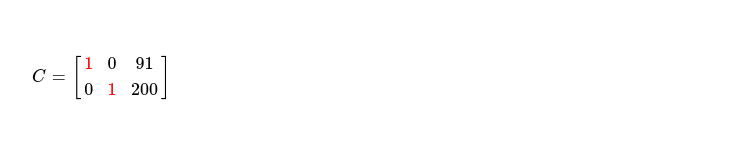Exact same row operations need to be performed on coded data matrix by two workers, which results into following. Note, these two worker threads are not synchronized.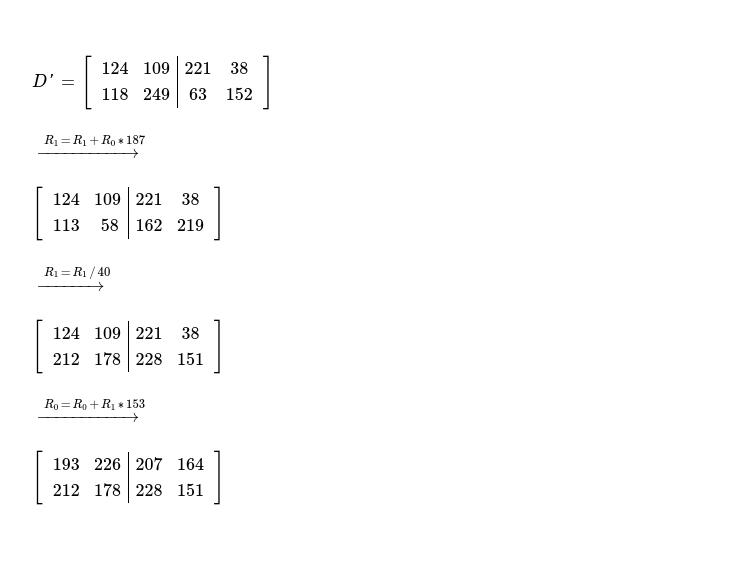After reception of third coded piece, coding coefficient matrix & coded data matrix looks like below. Again, aforementioned 3 steps to be performed for decoding data.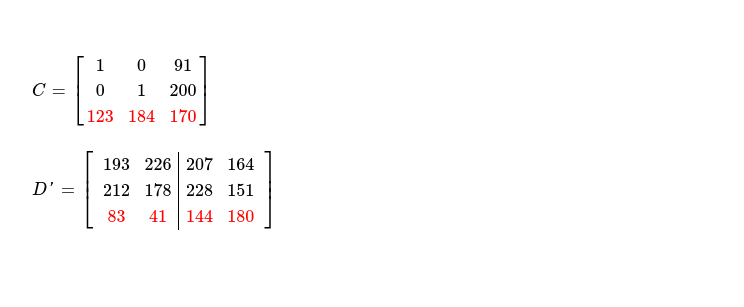For eliminating cells below pivots i.e. C & C, following row operations are performed. After following row operations i.e. completion of step 1, coding coefficient matrix looks as below.

1. C = C + C * C
2. C = C + C * C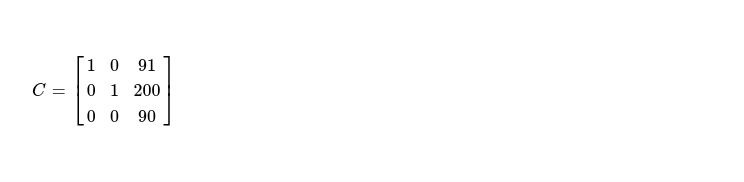As it turns out, pivot element C is non-zero i.e. third piece is linearly independent with other two pieces, I can proceed with step 2, where pivot elements are made 1.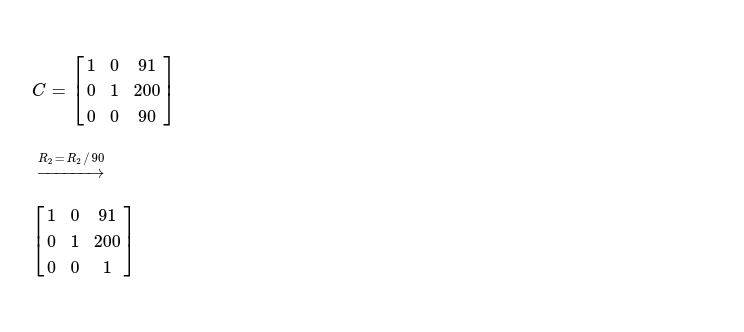And finally step 3, attempts to clean cells above pivot elements i.e. here C. For zeroing cells above C, following sets of row operations need to be performed, which results into below coding coefficient matrix --- N x N identity matrix, denoting all pieces required for decoding data are received, as rank of this matrix = 3 = generation size.

1. C = C + C * C
2. C = C + C * C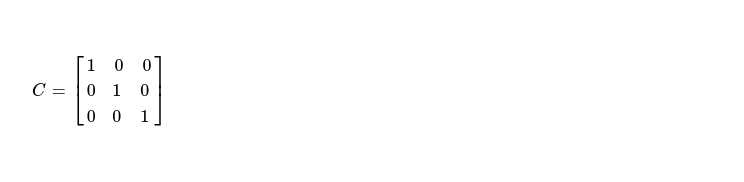For actually consuming decoded data, I need to wait for both worker threads to execute all pending row operations. After completion of all pending row operations in coded data matrix, original pieces are revealed --- ready for consumption by client application. Before worker threads stop, they must send one message to supervisor thread notifying completion of all tasks, in submatrix allocated to it.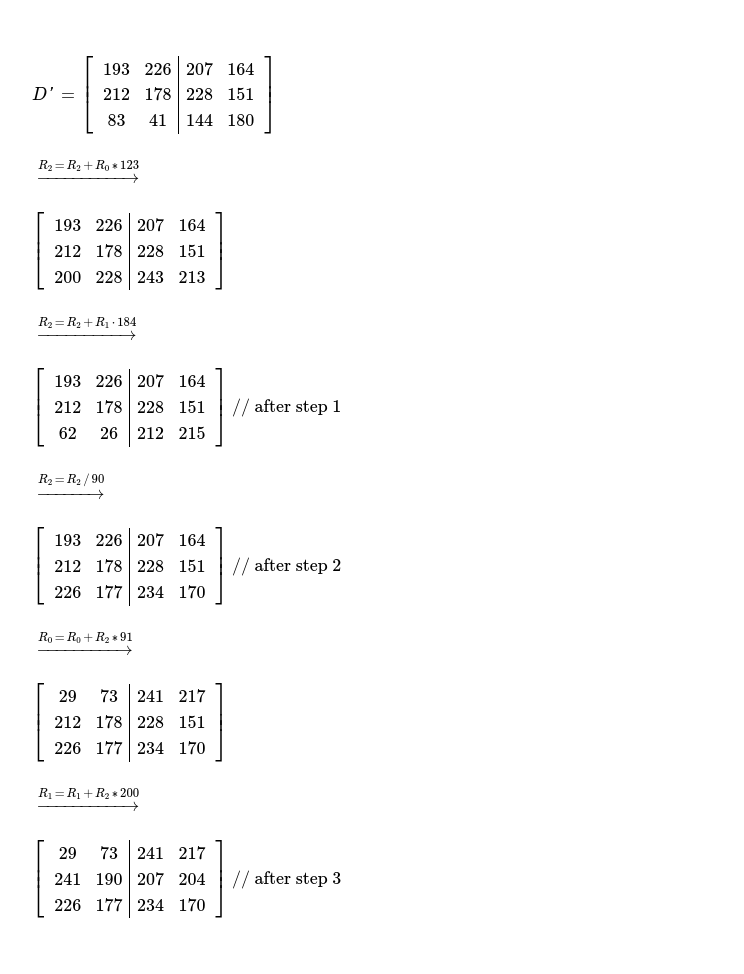This way Parallel RDPD is able to harness power of multiple CPU cores available in most of modern machines, for decoding Random Linear Network Coded data with minimal corridination among all employed threads. As worker threads are not synchronized, they're free to proceed at their own pace, except at very end of decoding supervisor expects to hear from all workers, notifying it of completion of execution of all pending elementary row operations.

How good is Parallel RDPD compared to single threaded decoding algorithm ? After I complete implementation of Parallel RDPD in kodr & benchmark it I'll find that out.

Have a great time !